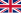Klosterneuburg, Austria

# Applied Mathematics, Mathematical Physics

Language: EnglishSubject area: mathematics and statistics
University website: www.ist.ac.at
Applied Mathematics
Applied mathematics is the application of mathematical methods by different fields such as science, engineering, business, computer science, and industry. Thus, applied mathematics is a combination of mathematical science and specialized knowledge. The term "applied mathematics" also describes the professional specialty in which mathematicians work on practical problems by formulating and studying mathematical models. In the past, practical applications have motivated the development of mathematical theories, which then became the subject of study in pure mathematics where abstract concepts are studied for their own sake. The activity of applied mathematics is thus intimately connected with research in pure mathematics.
Mathematical Physics
Mathematical physics refers to the development of mathematical methods for application to problems in physics. The Journal of Mathematical Physics defines the field as "the application of mathematics to problems in physics and the development of mathematical methods suitable for such applications and for the formulation of physical theories". It is a branch of applied mathematics, but deals with physical problems.
Mathematics
Mathematics (from Greek μάθημα máthēma, "knowledge, study, learning") is the study of such topics as quantity, structure, space, and change. It has no generally accepted definition.
Physics
Physics (from Ancient Greek: φυσική (ἐπιστήμη), translit. physikḗ (epistḗmē), lit. 'knowledge of nature', from φύσις phýsis "nature") is the natural science that studies matter and its motion and behavior through space and time and that studies the related entities of energy and force. Physics is one of the most fundamental scientific disciplines, and its main goal is to understand how the universe behaves.
Physics
I was an atheist, finding no reason to postulate the existence of any truths outside of mathematics, physics and chemistry. But then I went to medical school, and encountered life and death issues at the bedsides of my patients. Challenged by one of those patients, who asked "What do you believe, doctor?", I began searching for answers.
Francis Collins, a geneticist who led the U.S. government’s effort to decipher the human genome (DNA). cnn.com
Physics
As soon as we venture on the paths of the physicist, we learn to weigh and measure, to deal with time and space and mass and their related concepts, and to find more and more our knowledge expressed and our needs satisfied through the concept of number, as in the dreams of Plato and Pythagoras.
D'Arcy Wentworth Thompson, On Growth and Form (1917)
Physics
It is impossible, and it has always been impossible, to grasp the meaning of what we nowadays call physics independently of its mathematical form.
Jacob Klein, Greek Mathematical Thought and the Origin of Algebra (1968)
Shallow lakes have been greatly affected by increased concentrations of nitrogen and phosphorus from intensive agriculture and increased human populations. These key nutrients for plant growth enter the aquatic environment, changing clear water to turbid through a phenomenon called eutrophication.